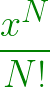# Program for sum of cosh(x) series upto Nth term

Given two numbers x and N, the task is to find the value of cosh(x) from the series upto N terms.

The expansion of cosh(x) is given below:

cosh(x) = 1 + x2/2! + x4/4! + …………

Examples:

```Input: x = 1, N = 5
Output: 1.54308035714

Input: x = 1, N = 10
Output: 1.54308063497
```

## Recommended: Please try your approach on {IDE} first, before moving on to the solution.

Approach:
The above series can be easily implemented using a factorial function and loops.

The nth term of the series is:Below is the implementation of the above approach:

## C++

 `// C++ program for ` `// the sum of cosh(x) series ` ` `  `#include ` `using` `namespace` `std; ` ` `  `// function to return the factorial of a number ` `int` `fact(``int` `n) ` `{ ` ` `  `    ``int` `i = 1, fac = 1; ` `    ``for` `(i = 1; i <= n; i++) ` `        ``fac = fac * i; ` ` `  `    ``return` `fac; ` `} ` ` `  `// function to return the sum of the series ` `double` `log_Expansion(``double` `x, ``int` `n) ` `{ ` ` `  `    ``double` `sum = 0; ` `    ``int` `i = 0; ` ` `  `    ``for` `(i = 0; i < n; i++) { ` ` `  `        ``sum = sum ` `              ``+ ``pow``(x, 2 * i) ` `                    ``/ fact(2 * i); ` `    ``} ` ` `  `    ``return` `sum; ` `} ` ` `  `// Driver code ` `int` `main() ` `{ ` `    ``double` `x = 1; ` `    ``int` `n = 10; ` `    ``cout << setprecision(12) ` `         ``<< log_Expansion(x, n) ` `         ``<< endl; ` ` `  `    ``return` `0; ` `} `

## Java

 `// Java program for the sum of ` `// cosh(x) series ` `import` `java.util.*; ` ` `  `class` `GFG ` `{ ` ` `  `// function to return the factorial of a number ` `static` `int` `fact(``int` `n) ` `{ ` `    ``int` `i = ``1``, fac = ``1``; ` `    ``for` `(i = ``1``; i <= n; i++) ` `        ``fac = fac * i; ` ` `  `    ``return` `fac; ` `} ` ` `  `// function to return the sum of the series ` `static` `double` `log_Expansion(``double` `x, ``int` `n) ` `{ ` `    ``double` `sum = ``0``; ` `    ``int` `i = ``0``; ` ` `  `    ``for` `(i = ``0``; i < n; i++)  ` `    ``{ ` `        ``sum = sum + Math.pow(x, ``2` `* i) /  ` `                           ``fact(``2` `* i); ` `    ``} ` ` `  `    ``return` `sum; ` `} ` ` `  `// Driver code ` `public` `static` `void` `main(String[] args)  ` `{ ` `    ``double` `x = ``1``; ` `    ``int` `n = ``10``; ` `    ``System.out.println(log_Expansion(x, n)); ` `} ` `} ` ` `  `// This code is contributed by 29AjayKumar `

## Python3

 `# Python3 program for the Sum of cosh(x) series ` ` `  `# function to return the factorial of a number ` `def` `fact(n): ` ` `  `    ``i, fac ``=` `1``, ``1` `    ``for` `i ``in` `range``(``1``, n ``+` `1``): ` `        ``fac ``=` `fac ``*` `i ` ` `  `    ``return` `fac ` ` `  `# function to return the Sum of the series ` `def` `log_Expansion(x, n): ` ` `  `    ``Sum` `=` `0` `    ``i ``=` `0` ` `  `    ``for` `i ``in` `range``(n): ` ` `  `        ``Sum` `=` `Sum` `+` `pow``(x, ``2` `*` `i) ``/` `fact(``2` `*` `i) ` ` `  `    ``return` `Sum` ` `  `# Driver code ` `x ``=` `1` `n ``=` `10` `print``(log_Expansion(x, n)) ` ` `  `# This code is contributed by Mohit Kumar `

## C#

 `// C# program for the sum of ` `// cosh(x) series ` `using` `System; ` ` `  `class` `GFG ` `{ ` ` `  `// function to return the  ` `// factorial of a number ` `static` `int` `fact(``int` `n) ` `{ ` `    ``int` `i = 1, fac = 1; ` `    ``for` `(i = 1; i <= n; i++) ` `        ``fac = fac * i; ` ` `  `    ``return` `fac; ` `} ` ` `  `// function to return the sum of the series ` `static` `double` `log_Expansion(``double` `x, ``int` `n) ` `{ ` `    ``double` `sum = 0; ` `    ``int` `i = 0; ` ` `  `    ``for` `(i = 0; i < n; i++)  ` `    ``{ ` `        ``sum = sum + Math.Pow(x, 2 * i) /  ` `                        ``fact(2 * i); ` `    ``} ` ` `  `    ``return` `sum; ` `} ` ` `  `// Driver code ` `public` `static` `void` `Main(String[] args)  ` `{ ` `    ``double` `x = 1; ` `    ``int` `n = 10; ` `    ``Console.WriteLine(log_Expansion(x, n)); ` `} ` `} ` ` `  `// This code is contributed by PrinciRaj1992 `

Output:

```1.54308063497
```

Don’t stop now and take your learning to the next level. Learn all the important concepts of Data Structures and Algorithms with the help of the most trusted course: DSA Self Paced. Become industry ready at a student-friendly price.

My Personal Notes arrow_drop_upCheck out this Author's contributed articles.

If you like GeeksforGeeks and would like to contribute, you can also write an article using contribute.geeksforgeeks.org or mail your article to contribute@geeksforgeeks.org. See your article appearing on the GeeksforGeeks main page and help other Geeks.

Please Improve this article if you find anything incorrect by clicking on the "Improve Article" button below.

Article Tags :
Practice Tags :

Be the First to upvote.

Please write to us at contribute@geeksforgeeks.org to report any issue with the above content.